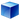# Cube Volume and Area Calculator

Length

Select Unit### How To Calculate Cube Volume?

Volume = lenght3 ,
ex : Cube have lenght = 15m Volume = 153 = 3375m3
.

### How To CalCulate Cube Area?

Cube Area = 6 * Lenght2,
ex : lenght = 14 , Cube Area = 6 * 142 = 1176m2.
the surface area of a cube = Lenght * Lenght , or Lenght2.